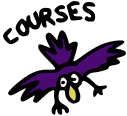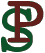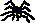Math 4680/5680, Topics in Logic and ComputationWinter 2012 Peter Selinger "Introduction to Mathematical Logic"

### Course Information

 See the Course Information Sheet.

### Announcements

 Final Exam (posted Apr 2). Our final exam will be on Monday, April 9, from 10-1 in Chase 319. Strike contingency plan (posted Mar 9). In case of a strike, you should continue doing course work: reading and assignments. Please see below for a week-by-week plan. Midterm (posted Feb 27). The midterm will be on Friday March 2 in class. Welcome to the course (posted Jan 4). Here, you will find up-to-date information, including announcements, homework assignments, any handouts, etc.

### Suggested Topics for Student Presentations

Here are some possible topics for graduate student presentations.
• Decidability. What does it mean for a function to be computable? What does it mean for a question to be decidable? Recursive and recursively enumerable sets.
• Analytic tableaux. Analytic tableaux are another proof system for first-order logic, well suited for automatic proof search. Reference: Raymond Smullyan, "First Order-Logic". Dover Publications, 1968, 1995.
• Non-standard models of arithmetic [taken]. Explore non-standard models of the natural numbers. In such models, there are "non-standard" numbers in addition to the "standard" numbers 0, 1, 2, etc. Reference: D. van Dalen, "Logic and Structure", Springer.
• Set theory [taken]. Explore the axioms of Zermelo–Fraenkel set theory. Set theory is a theory of first-order logic, with a single binary predicate symbol "∈", consisting of 7 axioms and 2 axiom schemas. Reference: Herbert B. Enderton, "Elements of Set Theory", Academic Press, 1977.
• Formal mathematics [taken]. Report on efforts to formalize real-world mathematics in computer-checkable form. Reference: Thomas C. Hales, "Formal Proof", Notices of the AMS, 55(11):1370--1380, December 2008.
• Type theory. Type theory is an alternative to set theory as a practical foundation of mathematics.
• Intuitionistic logic [taken]. Intuitionistic logic is concerned with constructive proofs: any proof of existence should yield an actual witness. Explore the laws of propositional intuitionistic logic and Kripke semantics. Reference: A. S. Troelstra and D. van Dalen, "Constructivism in mathematics: an introduction", North-Holland, 1988.
• Sequent calculus. Sequent calculus is an alternative proof system for first-order logic. It has a particularly attractive symmetry. Reference: Jean Gallier, "Constructive Logics. Part I: A Tutorial on Proof Systems and Typed lambda-Calculi". Theoretical Computer Science, 110(2), 249-239 (1993).

### Homework Assignments

 Homework 1, due Jan 23. 1.1 #2, 3; 1.2 #2, 5, 7, 10; 1.4 #2. Answers: PDF. Homework 2, due Feb 13. 1.5 #1, 4; 1.7 #12; 2.1 #1; 2.2 #2, 8, 11, 15. Answers: PDF. Homework 3, due Feb 27. 2.1 #2; 2.2 #1, 9, 14. Answers: PDF. Homework 4, due Mar 16. Lecture Notes 5, #1 (a)-(f), #2 (v): for each problem, prove the left-to-right implication, using your choice of Prawitz style or Fitch style natural deduction. Lecture Notes 5, # 3 (d), (j), (l). Handout 6, p.114 #1, 2. Answers: PDF.

### Contingency Plan

 In case of a strike, you should continue doing course work (reading and assignments). No homework will be due during a strike, but after a strike, the end of term will likely be accelerated. Here is a week-by-week contingency plan for what to study: March 12-16: Reading: 2.6. Homework 2.5 #8, 2.6 #2, 4, 7, 8. March 19-23: Reading: 2.8, 3.0. Homework 2.8 #1, 5. March 26-30: Reading: 3.1, 3.3, 3.4. Homework 3.1 #4, 5, 6. April 2-4: Reading: 3.5. Homework 3.3 #1, 3, 10.

### Course Notes

 Lecture Notes 1 (posted Jan 15) [pdf]: The Language of Sentential Logic. Lecture Notes 2 (posted Jan 15) [pdf]: Truth, Proofs, Soundness, and Completeness for Sentential Logic. Lecture Notes 3 (posted Feb 17) [pdf]: The Language of First-Order Logic. Lecture Notes 4 (posted Feb 17) [pdf]: Soundness, Completeness, and Consequences for First-Order Logic. Lecture Notes 5 (posted Feb 17) [pdf]: Fitch style natural deduction.

### Other Handouts

To Peter Selinger's Homepage: